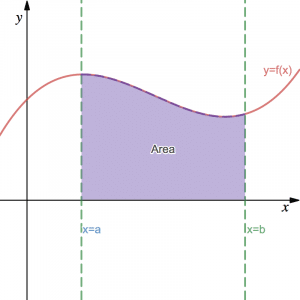# Integration – reversing differentiation & finding areas

## Integration

Integration (which, like differentiation, is part of calculus) is the process we use to reverse differentiation and/or find areas beneath curves. Recall that, during GCSE Maths, we saw how to find the area beneath some straight line graphs (area under velocity-time graph). At A-Level, we learn how to do this for curves.Indefinite and Definite Integration of basic polynomial functions, as well as the fundamental theorem of calculus, are taught in the first year of A-Level Maths. In the second year, we will learn multiple methods to integrate more complicated functions as listed below. We also learn how to solve differential equations.